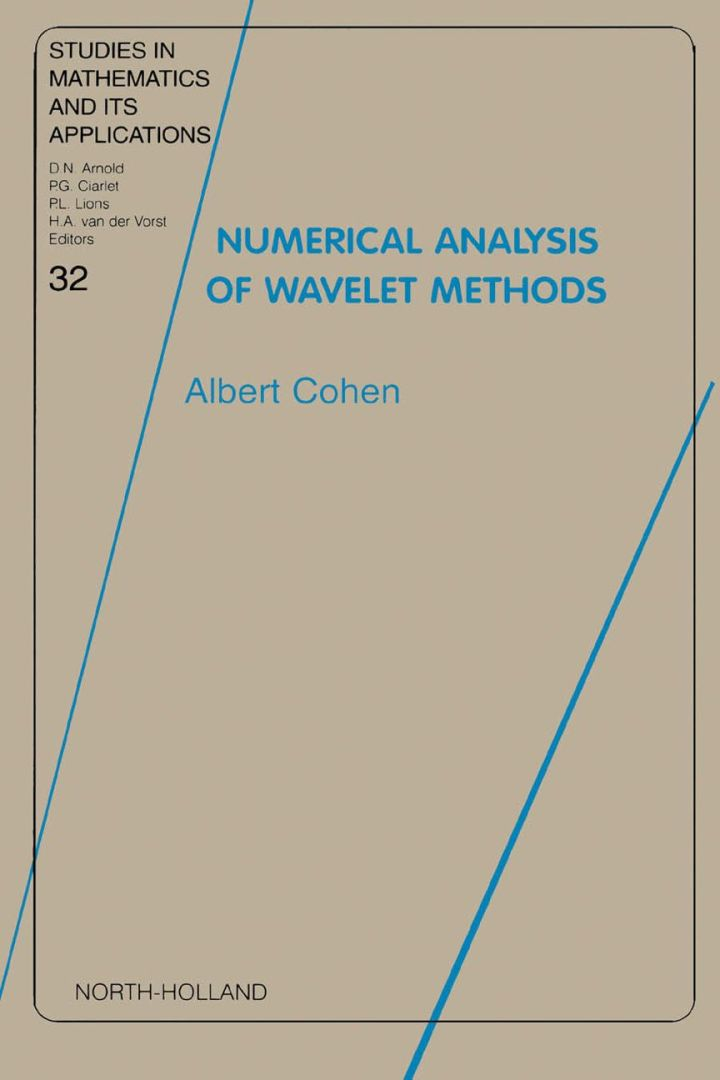# Numerical Analysis of Wavelet Methods

## Numerical Analysis of Wavelet Methods

By Cohen, A.

### Rent or Buy eTextbook

Expires on Apr 19th, 2022
\$137

Publisher List Price: \$139.99
Savings: \$2.99

Since their introduction in the 1980's, wavelets have become a powerful tool in mathematical analysis, with applications such as image compression, statistical estimation and numerical simulation of partial differential equations. One of their main attractive features is the ability to accurately represent fairly general functions with a small number of adaptively chosen wavelet coefficients, as well as to characterize the smoothness of such functions from the numerical behaviour of these coefficients. The theoretical pillar that underlies such properties involves approximation theory and function spaces, and plays a pivotal role in the analysis of wavelet-based numerical methods.
This book offers a self-contained treatment of wavelets, which includes this theoretical pillar and it applications to the numerical treatment of partial differential equations. Its key features are:

1. Self-contained introduction to wavelet bases and related numerical algorithms, from the simplest examples to the most numerically useful general constructions.

2. Full treatment of the theoretical foundations that are crucial for the analysis
of wavelets and other related multiscale methods : function spaces, linear and nonlinear approximation, interpolation theory.

3. Applications of these concepts to the numerical treatment of partial differential equations : multilevel preconditioning, sparse approximations of differential and integral operators, adaptive discretization strategies.

Subject: Mathematics & Statistics -> Mathematics -> Mathematics GeneralPublisher: Elsevier S & T 04/2003
Imprint: JAI Press
Language: English
Length: 354 pages

ISBN 10: 0444511245
ISBN 13: 9780444511249
Print ISBN: 9780444511249

Live Chats